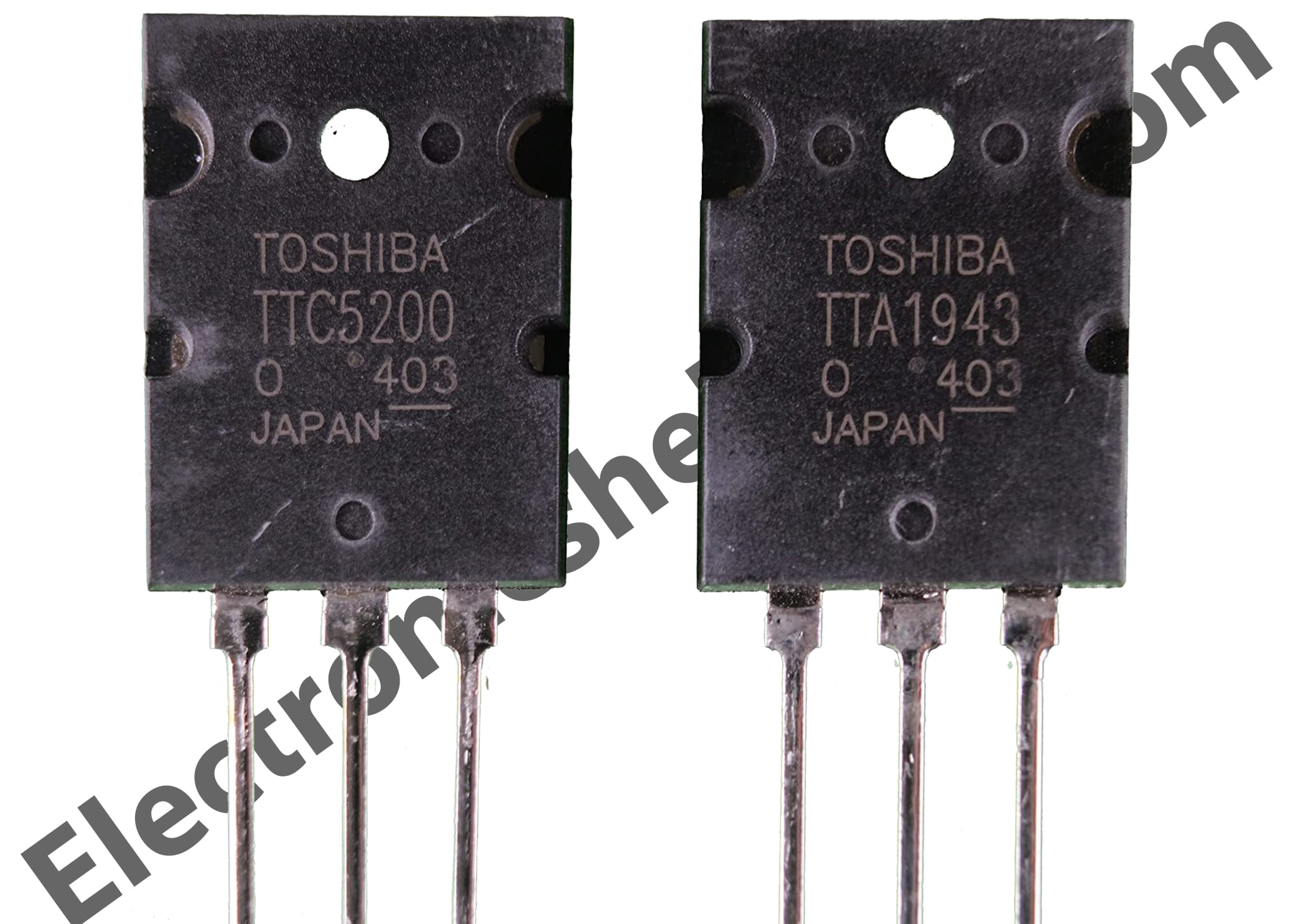# amplifier circuit diagram using transistor

## amplifier circuit diagram using transistor:

This is a 200 watts transistor amplifier circuit diagram using the transistor. here used tta1943 and ttc5200 transistor. This pair of transistors can make 100 watts. here used 4 transistors. 2 transistors can make 100 watts. but here used 4 transistors. so it will make 200 watts. here needs dual power. positive negative and ground voltage.It will be 50X6=300 watts but this circuit can make 200 watts maximum. for this cause, we have to use a maximum of 200 watts of voltage and amperes. like 50 voltage and 4 amperes. or 40 voltage and 5 amperes. we can’t use over-voltage according to circuit capacity. we have to be under 200 watts. otherwise, the circuit will be burnt. we need dual voltage. Negative positive and the ground voltage. need a center tap transformer to make dual voltage. always we have to use a strong voltage to get good watts.how to use diode and capacitorOn our website, you can get many important circuit diagrams. not only amplifier but also invertervoltage controller. transistor amplifier. STK amplifier. Class-D amplifier. bass circuit/ low pass filter

Please always stay with us to get circuit diagrams. and give us advice at Electronics help care